Methods and formulas for Autocorrelation

Select the method or formula of your choice.

ACF

The graphs for the autocorrelation function (ACF) of the ARIMA residuals include lines that represent the significance limits. Values that extend beyond the significance limits are statistically significant at approximately α = 0.05, and show evidence that the autocorrelation does not equal zero.

Notation

TermDescription
k lag; k = 1, 2,...
xt value of x at row tmean of x
n number of observations in the series

Standard error of ACF

Notation

TermDescription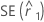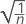k lag; k = 1, 2, ...
n number of observations in the series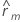autocorrelation of lag m

t-statistics

Notation

TermDescription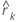autocorrelation at lag k; k = 1, 2, ...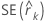standard error of the autocorrelation at lag k

Significance limits

Formula

Upper limit at lag k = t n−1; 0.975 × SE(rk )

Lower limit at lag k = t n−1; 0.025 × SE(rk )

Notation

TermDescription
SE(rk ) standard error of the autocorrelation at lag k
t n-1; 0.975 97.5th percentile of the t distribution with n – 1 degrees of freedom
t n-1; 0.025 2.5th percentile of the t distribution with n – 1 degrees of freedom

Ljung-Box Q statistic

Notation

TermDescription
n number of observations in a seriesestimated autocorrelation at lag m; m = 1, 2, ..., k
klag; k = 1, 2, ...
By using this site you agree to the use of cookies for analytics and personalized content.  Read our policy